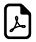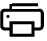# Order of operations PEMDAS Chart

The Order of operations is a collection of rules that define which procedures to perform first in order to evaluate a given mathematical expression.## Order of operations Chart

MnemonicExpansionOrder of OperationsExample
PEMDAS Please Excuse My Dear Aunt Sally which do you do first? = (2 + 7 ) + 4 ? 3 - 10
P P stands for Parentheses First Do Parenthesis:
= 9 + 4 ? 3 - 10
E E stands for Exponents Second N/A for this example
M M stands for Multiply Third Do Multiplication
= 9 + 12 - 10
D D stands for Division Fourth N/A for this example
A A stands for Add Fifth Do Addition
= 21 - 10
S S stands for Subtraction Sixth Do Subraction
= 11